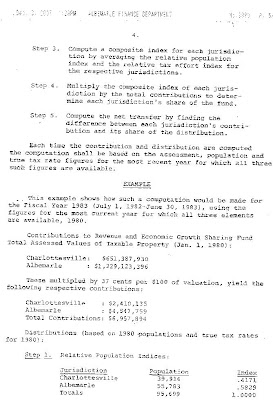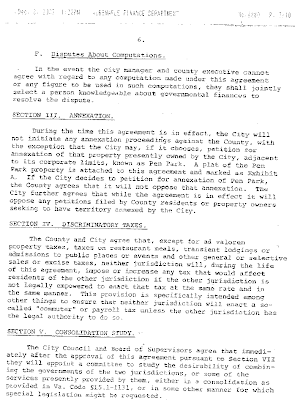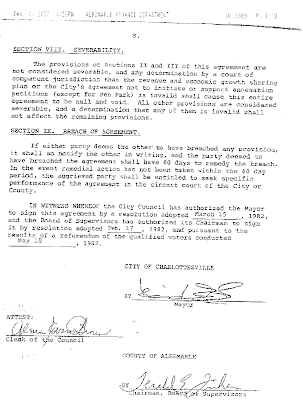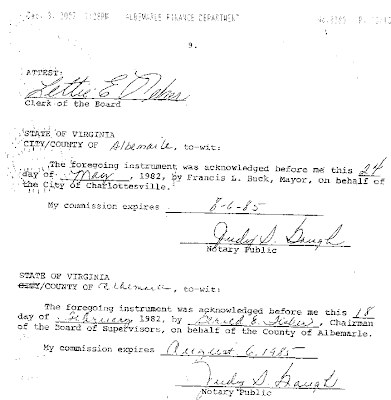Tuesday, March 04, 2008

1982 Revenue Sharing agreement published

Charlottesville, Va.- Below are all 9 pages of the actual 1982 Revenue Sharing agreement between the independent city of Charlottesville and the surrounding county of Albemarle. There's also a table of transfer payments since the agreement took effect. Below that is my first attempt to make the formula more meaningful. Feel free to add a comment or correction. Thanks to Joe Thomas of WCHV for emailing the document to me and thanks to Hank Martin who made the document available to Joe.Historic Preservation Guide, Charlottesville, Virginia July 1980, Page 6.The Formula

Information needed:

- Population of the city and county as determined by US Census, and by the Tayloe Murphy Institute of the University of Virginia in years between censuses.

- True real property tax rates for city and county as determined by Virginia Department of Taxation.

- Total Assessed Value of Taxable Property for city and county to find Total Contribution to revenue and economic growth sharing fund.

a=city and b=county

Step 1: Population Index

PIa = Pa / (Pa + Pb)
PIb = Pb / (Pa + Pb)

Step 2: Tax Index…T=Tax Rate

TIa = Ta / (Ta + Tb)
TIb = Tb / (Ta + Tb)

Step 3: Composite Index

CIa = (PIa + TIa) / 2
CIb = (PIb + TIb) / 2

Step 4: Contribution to Fund…0.0037 is a valuation factor (37cents per \$100 assessed value)

Ca = (0.0037)(Total Value of Taxable Property in city)
Cb = (0.0037)( “ “ county)

Step 5: Distribution

Da = (CIa)(Ca + Cb)
Db = (CIb)(Ca + Cb)

Step 6: Net Transfer

Net = Da – Ca = Db – Cb

Here’s the first example given for Jan. 31, 1983 initial payment.

Pa = 39,916
Pb = 55,783
Ta = 0.91510
Tb = 0.49848

CIa = ½ [ (Pa / (Pa+Pb)) + (Ta / (Ta+Tb)) ]
CIb = ½ [ (Pb / (Pa+Pb)) + (Tb / (Ta+Tb)) ]

Ca = (0.0037)(\$651,387,930) = 2,410,135
Cb = (0.0037)(\$1,229,123,396) = 4,547,759

Total Contributions C = (Ca+Cb)=6,957,894

Distribution

Da=(CIa)(Ca+Cb)= (0.5323)(6,957,894) = 3,703,687
Db=(CIb)(Ca+Cb)= (0.4677)(6,957,894) = 3,254,207

Net Transfer
NET = Da – Ca = Db – Cb

NETa = Da–Ca= 3,703,687 – 2,410,135 = +\$1,293,552
NETb = Db–Cb= 3,254,552 – 4,547,759 = –\$1,293,552

(+) is transfer to you and (–) is transfer from you

NETa= (CIa)(Ca+Cb) – (0.0037)(total assessed property value for city)
NETb= (CIb)(Ca+Cb) – (0.0037)( “ “ county)